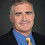## Monday, October 17, 2011

The last few times I've taught undergraduate theory I cover the PCP theorem. It's not complicated if you state it the right way:

PCP Theorem: For any constant α > 7/8, there is a polynomial-time computable function f mapping 3-CNFs to 3-CNFs such that for all formula φ,
• If φ is satisfiable then f(φ) is satisfiable.
• If φ is not satisfiable then every assignment to f(φ) satisfies at most an α-fraction of the clauses.
I point out to the class you can satisfy 7/8 of the clauses by just choosing a random assignment.

Also I show how the PCP theorem gives some (weak) approximation bounds for Clique. For each variable in each clause of f(φ) create a node of a graph and connect two nodes as long as they aren't in the same clause or connect a node representing a variable with one representing its negation. That gets you an 8/7-ε approximation lower bound for clique. I give showing a constant approximation lower bound for Vertex cover as a homework assignment.

I don't even try to give an idea of the proof of the PCP theorem. I just say it would take an entire graduate class to cover the proof in its full detail. That's probably a lie, one of our ten-week quarter-long classes is not enough time to prove the very strong version of the PCP theorem stated above.

1.In all seriousness: would it really not be possible to give the proof in a graduate seminar? Assuming the students had taken a graduate complexity class beforehand, I think covering the proof, in full detail, could be done in < 10 1-hour lectures.

Moreover, there have already been several classes devoted to the PCP theorem. =)

2.Lance, do you explain what the above statement has to do with the checking of proofs? (I'm assuming you tell your students what PCP stands for.)

Amit C

3.The assignment is the proof you can check with three queries by picking a random clause. I briefly mention this in class but it's the application to approximation that is more important.

4.5.